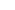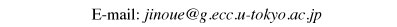Example of mcmctree analysis 〜Beginning guide〜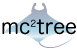Oct. 29, 2015 Jun Inoue

MCMCTREE is a phylogenetic program for Bayesian estimation of species divergence times using soft fossil constraints under various molecular clock models. This is part of the PAML package. In this tutorial I will analyze an easy example modified from dataset of Inoue et al. (2010). Here we conduct a commonly used time estimation method, "Approximate Likelihood Method", for the datasets including more than 10 species.

I am assuming that you have some basic knowledge of command line in Windows or Unix system in Linux/MacOS．

Make sure you are using the latest version of PAML. Now I am using 4.8a [Sept. 2015]．

Here
is an explanation by slide.

Japanese language version is here.

 Use of Bayesian method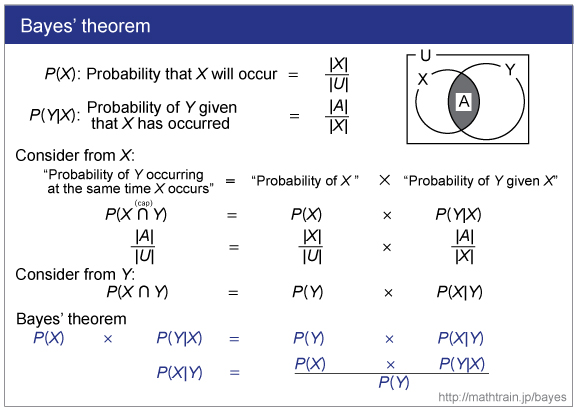See this site.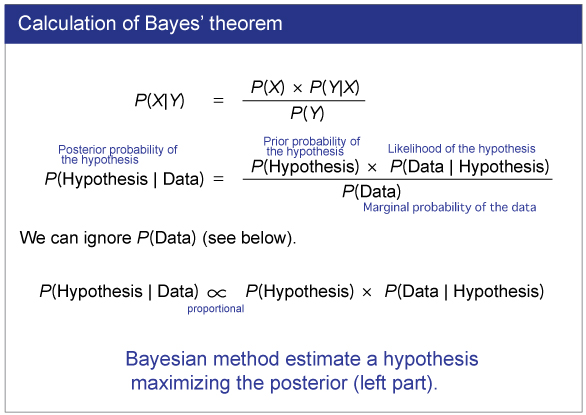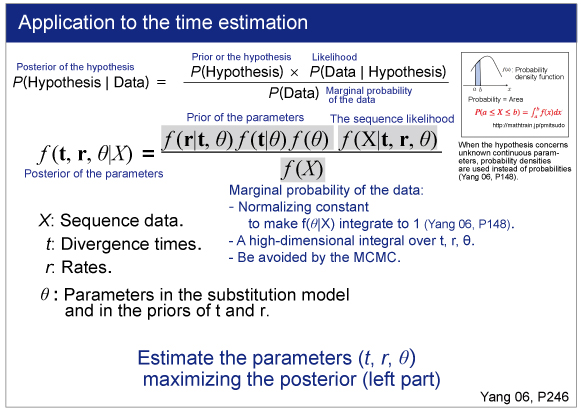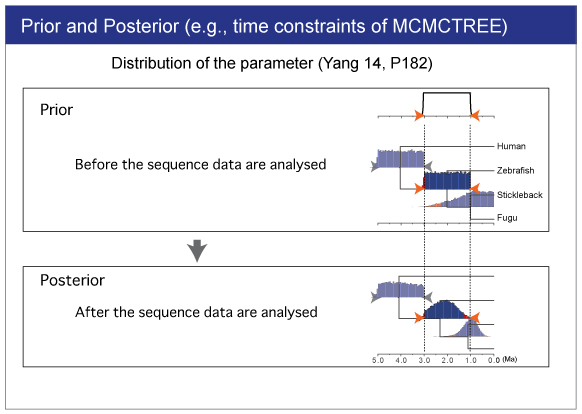MCMCTREE analysis
• Command line．
(Windows: command prompt; Mac: terminal)．
• Topology is fixed．
• Fossil constraints needs to be researched．
• DNA/AA sequence data can be analyzed．
• Genome data can be analyzed．

 Preparation of example and softwares

Download & install example and softwares. If you cannot download them, use the softwares within the mcmctreeEasyExam.tar.gz.

Example
Download the following link.
mcmctreeEasyExam.tar.gz

In this manual, we use the data saved in the mcmctreeEasyExam/examples folder.

Unzipping program [Windows only]
For Windows, we need Lhaplus for the compression of .tar. file．
• Download & install the unzipping program Lhaplus (free).
• Unzip．Drag & drop the downloaded mcmctreeEasyExam.tar.tar (or mcmctreeEasyExam.tar) file，to the Lhaplus alias (desktop).
• mcmctreeEasyExam folder will appear in the desktop.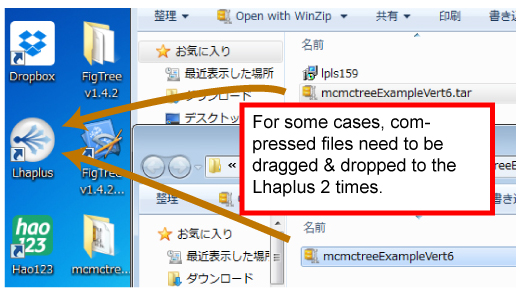Change the property of folder
[Windows]
Show extensions (.exe)．Uncheck the following．

Control panel> Option of folder and search > Display > Do not show the extension (登録されている拡張子は表示しない)

Change the property of the folder and included files. Right click the mcmctreeEasyExam/examples folder and uncheck the following:

Property > Read only

[Mac]
Select examples folder by cursor and type command+I to see information. Change the access right to "Read/ Wright".

Display of textile
[Windows]
As a trial, let's open a file with editor (Notepad). Open mcmctreeEasyExam/examples　folder．Right click baseml.ctl and select the following:

Open with Notepad

(サクラエディタ [Japanese editor])

[Mac]
Text Wrangler is useful.

Download and preparation of analysis softwares
MCMCTREE is part of the PAML package. Download the PAML package from the following link http://abacus.gene.ucl.ac.uk/software/paml.html. The latest version is paml4.8a.tgz (Sep. 2015).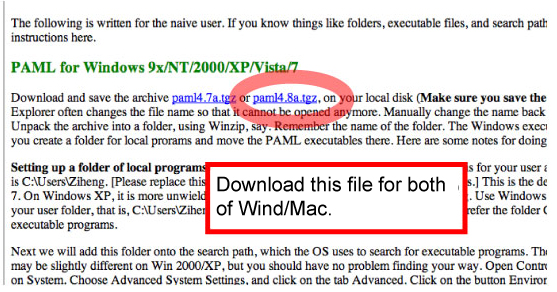[Windows]
Copy & paste the following 2 programs from pamlXX/bin to mcmctreeEasyExam/examples folder.

baseml.exe
mcmctree.exe

These programs can be used without compilation.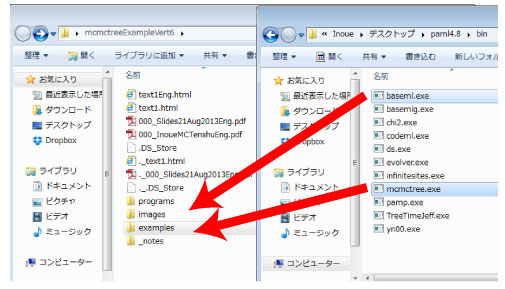[Mac]
Unzip the downloaded pamlXX.tar.gz file by double click．Enter the pamlXX/src folder from Terminal．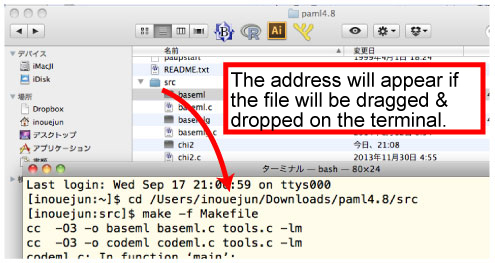Then compile the programs.

> make -f Makefile

The compilation will make 2 programs, BASEML and MCMCTREE, in src folder. Copy & paste these 2 programs to mcmctreeEasyExam/examples folder.
Caution: If you cannot find "make" command in your Mac, install the X code (

Operation check

 [Windows] From command prompt, enter mcmctreeEasyExam/examples folder. The command prompt can be found in the following:コマンドプロンプトの場所は， Program > Accessory > Command promptType the following command．

cd xxxx/xxxx/mcmctreeEasyExam/examples

Copy the address (underlined) as follows.
" dir" command show the content of the folder.

dir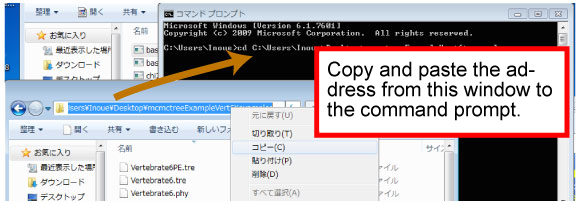Run BASEML．Type the following command in the command prompt．

> baseml baseml.ctl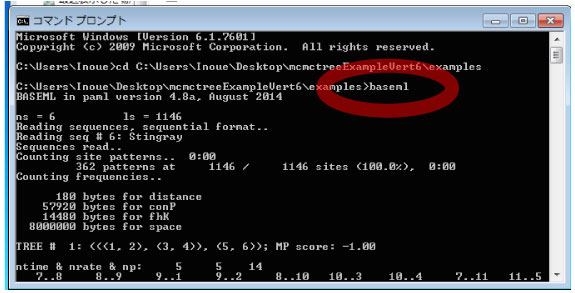[Mac]

Enter the mcmctreeEasyExam/examples folder and type the following command.

> ./baseml baseml.ctl

 Infiles
Check the following infiles in your mcmctreeEasyExam/examples folder. If you cannot overwrite, uncheck "read only" from wright click > property (Windows)．

baseml.ctl
Control file for baseml．

mcmctree.ctl
Control file for MCMCTREE．

Vertebrate6.phy
Sequence file. This file contains a nucleotide alignment of 6 vertebrate species.

Vertebrate6PE.tre and Vertebrate6.tre
Tree file．This file contains constrained tree as the Newick format.

Display of tree
[Windows & Mac]
Make sure the topology and constraints of Vertebrate6PE.tre and Vertebrate6.tre (Newick format trees) using FigTree. You can draw the trees as follows: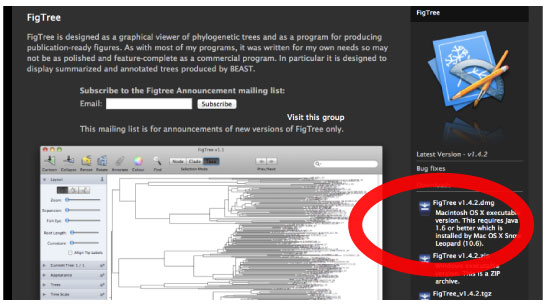[Windows & Mac]
Check the constrained tree by FigTree．Open Vertebrate6PE.tre file by Drag & drop.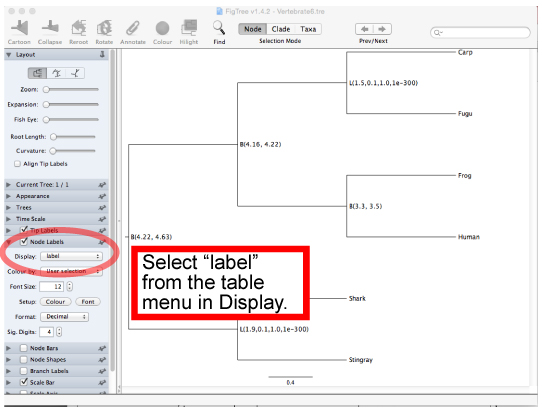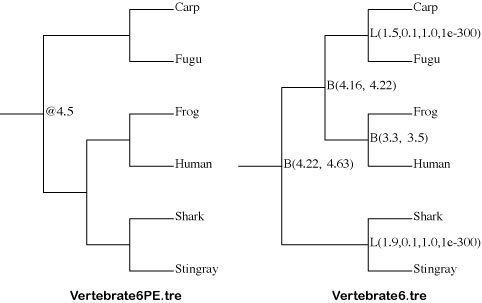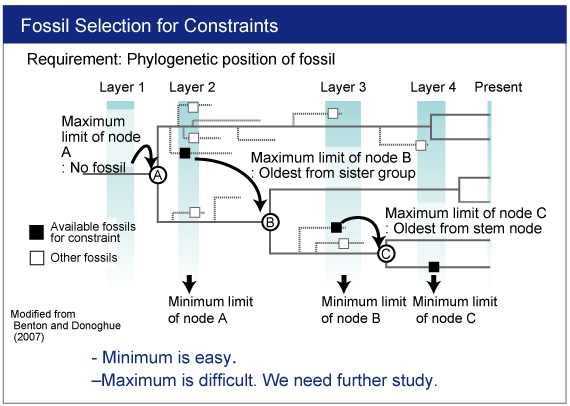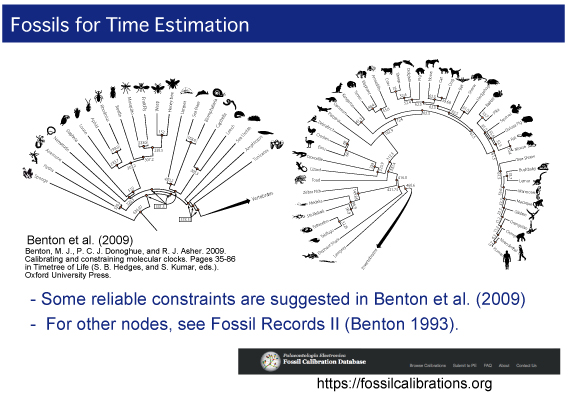Workflow

The approximate likelihood method consists of 2 steps (3 steps including preparation). We conduct each analysis as different job.

0. Rough Estimation of Substitution Rate
1. Estimation of Gradient and Hessian of Branch Lengths
2. Estimation of Time and Rate by MCMC Analysis

Caution： For Windows, sometimes we need to change "attribute" of example directory by right click. Then unlock "read only"．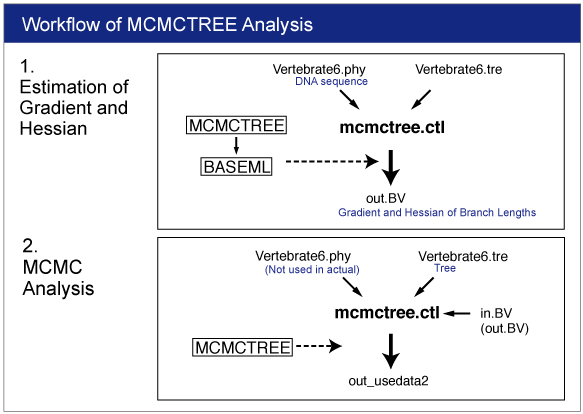0. Rough Estimation of Substitution Rate
We will estimate overall substitution rate in this dataset. This estimates will be used in rgene_gamma setting in mcmctree.ctl later.

Move into the mcmctreeEasyExam/examples directory by command prompt (Win) or terminal (Mac).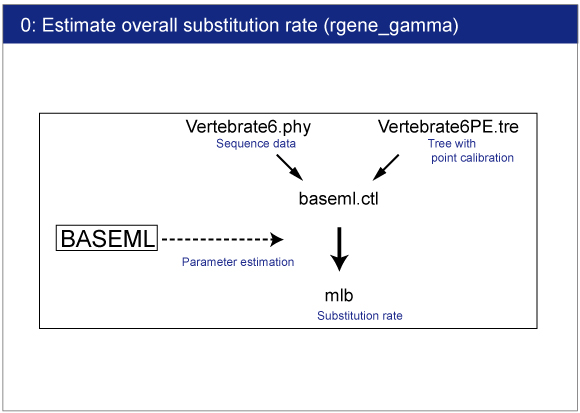## Infiles Open baseml.ctl by your editor．Sequence file (Vertebrate6.phy) and tree file (Vertebrate6PE.tre) names are written．GTR+G model (model = 7) will be used for rate estimation．Strict clock model (clock = 1) is used because of quick estimation．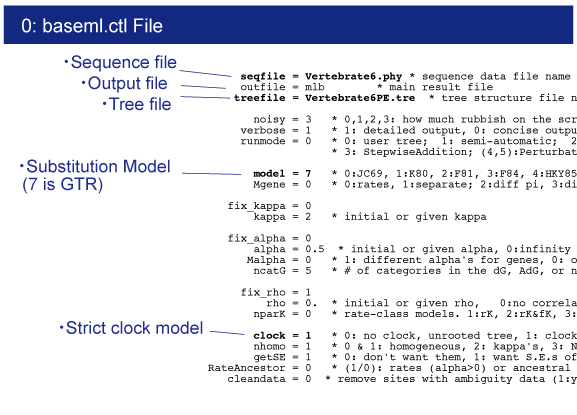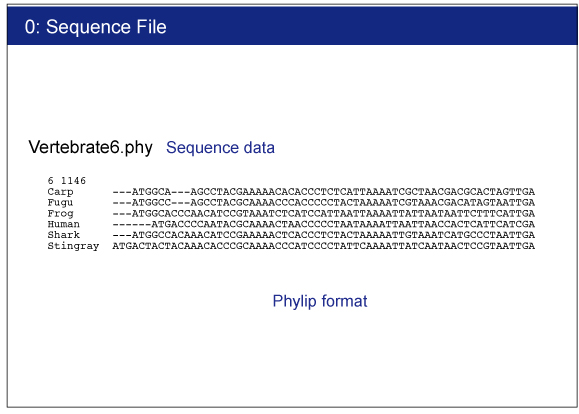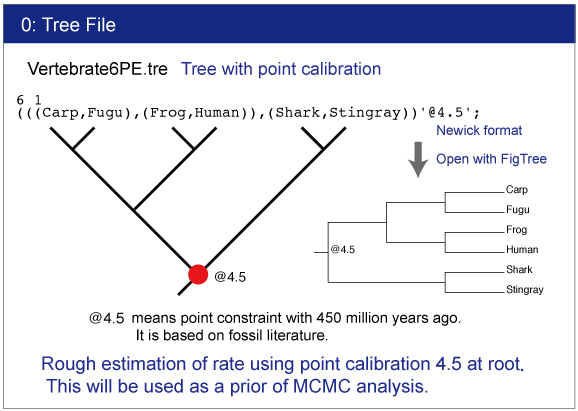Execution of BASEML
[Windows]
From command prompt, type,

## > baseml baseml.ctl[Mac] From terminal, type,

> ./baseml baseml.ctl

## If the control file is named as baseml.ctl, baseml read it automatically without specifying.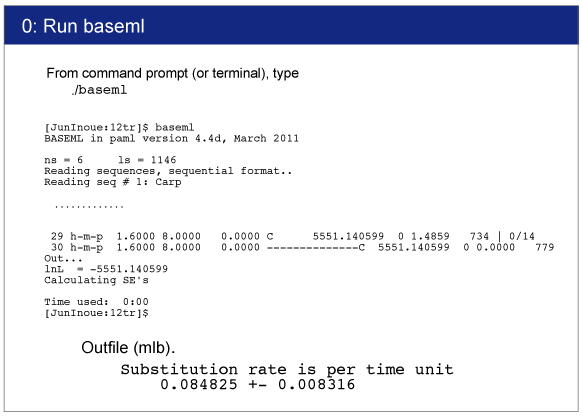## Result Result will be saved in mlb file．You can find the following result in mlb file:

Substitution rate is per time unit
0.084825 +- 0.008316

The exact value would differ depending on the program version. This value will be used in mcmctree.ctl file.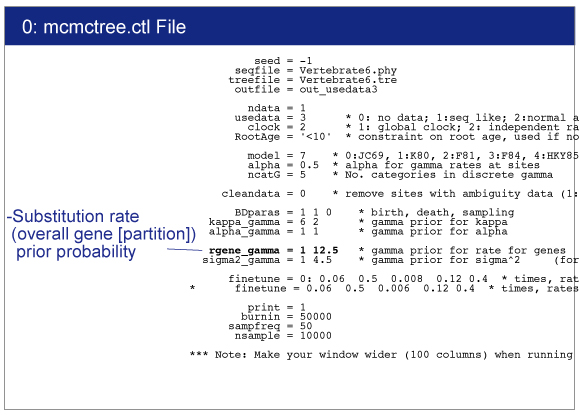1. Estimation of Gradient and Hessian of Branch Lengths
The branch lengths are estimated by maximum likelihood together with the gradient and Hessian (i.e. vector of first derivatives and matrix of second derivatives) of the likelihood function at the maximum likelihood estimates. The gradient and Hessian contain information about the curvature of the likelihood surface (dos Reis and Yang, 2013, P10)．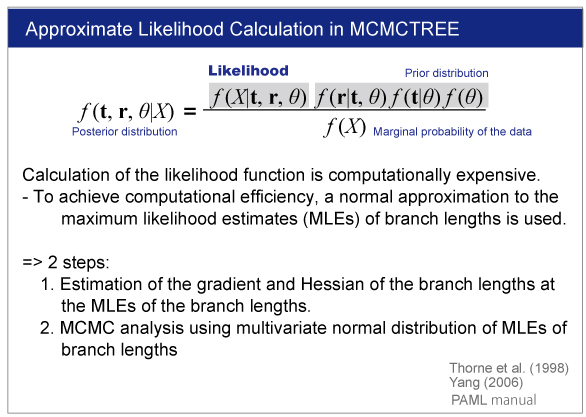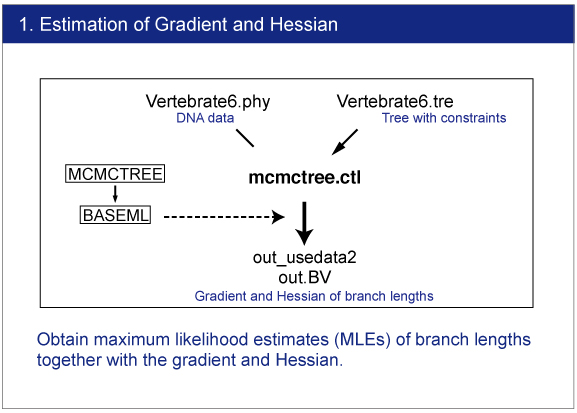Infiles
Open mcmctree.ctl file by your editor and make sure the following line:

usedata = 3

This option is for the estimation of the maximum likelihood estimates (MLE) of branch lengths, gradient (g), and Hessian (H).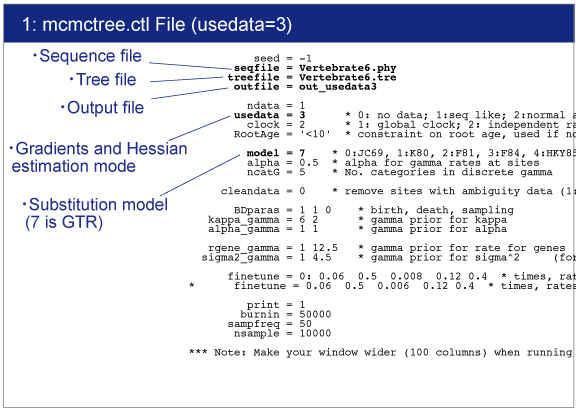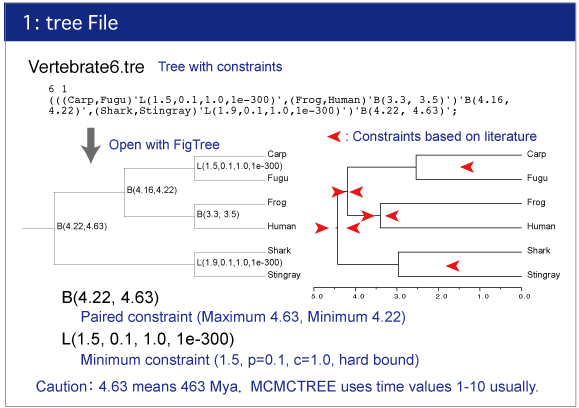Execution of MCMCTREE

Type,

> mcmctree mcmctree.ctl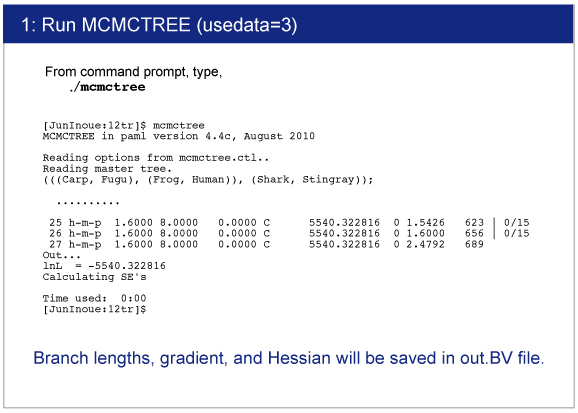Result
The estimated gradient and Hessian will be saved in out.BV file.2. Estimation of Time and Rate by MCMC Analysis
We will estimate divergence times by Bayesian analysis.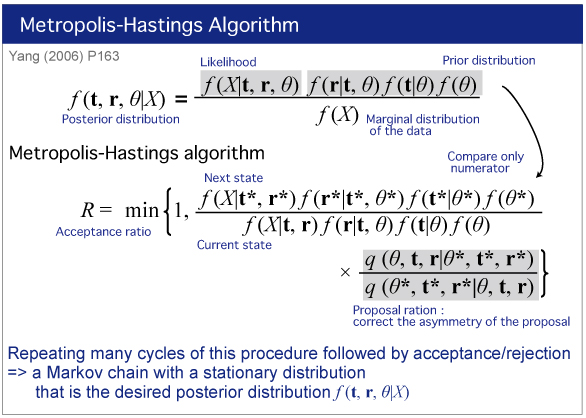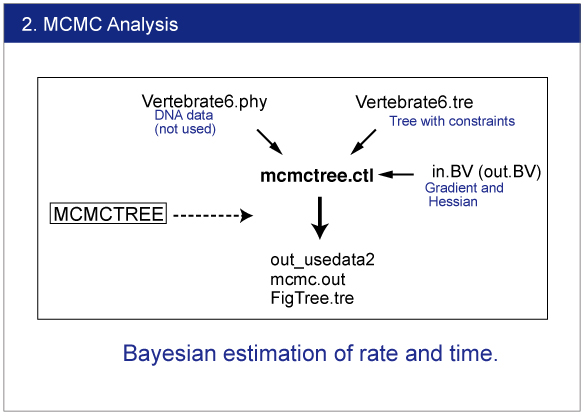Infiles
Change the name "out.BV" to "in.BV"．

Open mcmctree.ctl file and change the usedata option as follows:

usedata = 2

This option will start the MCMC analysis using Approximate Likelihood Calculation. Also change the name of output file as follows:

outfile = out_usedata2rgene_gamma
rgene_gamma specifies the prior distribution of overall substitution rate μ with assuming the gamma distribution. Here we need to specify the shape and scale parameters of the gamma distribution.

The gamma distribution is described by the shape parameter (α) and scale parameter (β)．Let m be the mean and s be the standard deviation of the gamma distribution. These are related as follows:
a = (m/s)^2
b = m/s^2.
For example, μ is 2/2 =1，then it means 1 change/site/time unit．If time unit is 100 MY，the overall substitution rate is 10^-8 substitution/site/year．
According to the rough estimation using BASEML (see above), we will use m = 0.08 and s = 0.08．The shape parameter α is
α = (0.08/0.08)^2
= 1.
And the scale parameter is
β = 0.08/0.08^2
=12.5.
So in the control file, we specify rgene_gamma as follows.

rgene_gamma = 1 12.5

* When the data constains multiple loci (e.g., ndata = 4), specify the parametes in the gamma-Dirichlet prior. See MCMCTREE manual (Sept. 2015).

sigma2_gamma

sigma2_gamma specifies how variable the rates are across branches. We specify the shape and scale parameters (α and β) in the gamma prior for parameter σ^2. In the control file, we set

sigma2_gamma = 1 4.5 .

See the PAML manual for detail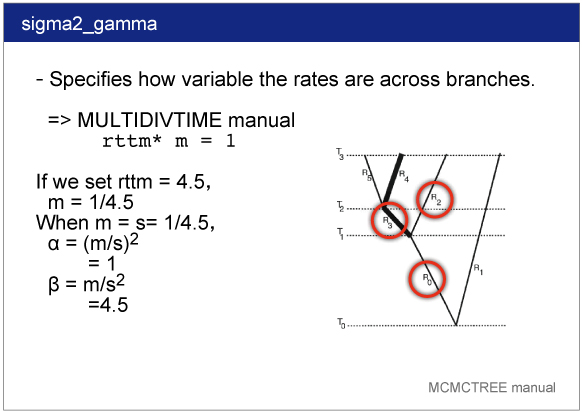Execution of MCMCTREE

> mcmctree mcmctree.ctl

After you start MCMCTREE, check acceptance proportion on your screen．Ideally they ranged around 30% (20〜40% is better, 15〜70% is acceptable)．

finetune = 1: 0.06 0.5 0.008 0.12 0.4 * times, rates, mixing, paras, RateParas

If the acceptance proportion are not stable, change "finetune = 1" to "finetune = 0" and modify the finetune values manually．In this case, you need to quit analysis using "Ctl+C". See the manual for detail.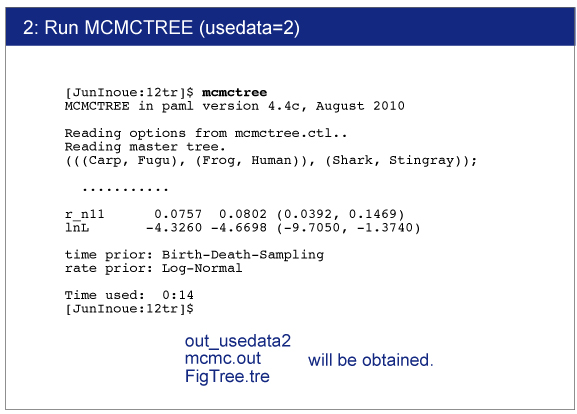Result
The result will be saved in out_usedata2 file．You can find the estimated time and 95% confidence interval for each node.The raw data is saved in mcmc.out file.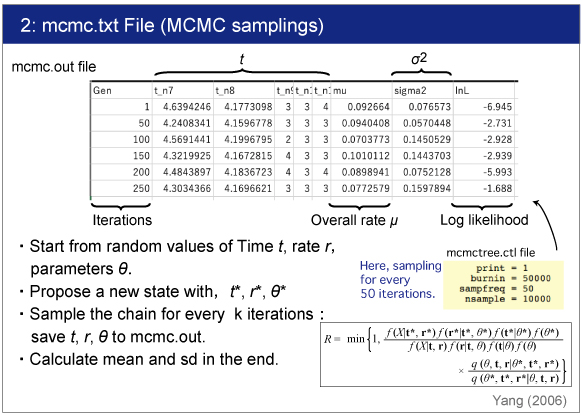3. Figure Making
FigTree.tre (output file of MCMCTREE program) can be used for the infile of FigTree.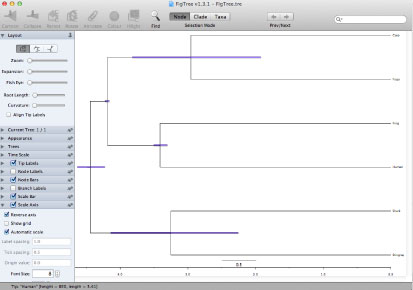↓ Add constraints by graphics editor (e.g., Illustrator).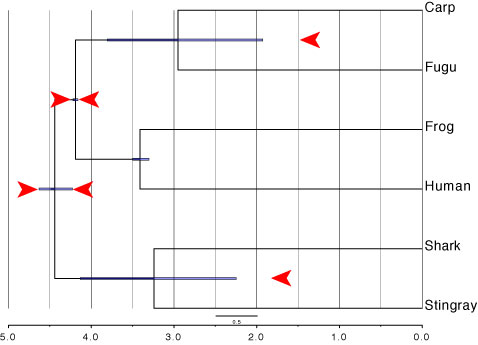For more advanced analysis , see the following instruction.
(A step-by-step manual for MCMCTREE with an example dataset).

References
dos Reis, M., Yang, Z. 2013. MCMCTREE tutorials. http://abacus.gene.ucl.ac.uk/software/MCMCtree.Tutorials.pdf

Holder, M. Bayesian Phylogenetics. http://phylo.bio.ku.edu/slides/BIOL848-BayesIntro.pdf

Thorne, J. L., H. Kishino, and I. S. Painter. 1998. Estimating the rate of evolution of the rate of molecular evolution. Molecular Biology and Evolution 15:1647–1657.

Yang, Z. (2006). Computational Molecular Evolution. Oxford, Oxford University Press.